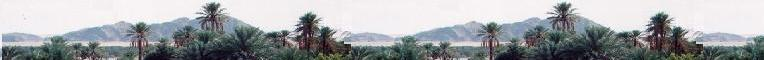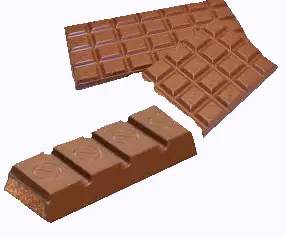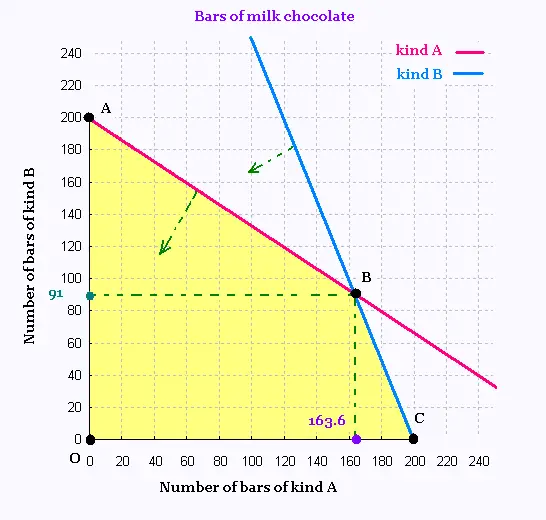# Mathematics 2: Linear optimization Examples

### ChocolateA company manufactures two kinds of chocolate bars with two principal ingredients: chocolate and milk.

Kind A : bar of 100 g chocolate and 25 g milk, which costs 4.00 $. Kind B : bar of 150 g chocolate and 10 g milk, which costs 6.00$.

The available quantities of chocolate and milk are 30 kg and 5 kg respectively.

How many bars of each kind the company would produce to maximize its profit ?

Set the unknown:

x is the number of bars of kind A
y is the number of bars of kind B

kind A : 100 x g of chocolate and 25 x g of milk
kind B : 150 y g of chocolate and 10 y g of milk

Thus

100 x + 150 y g of chocolate, and
25 x + 10 y g of milk.

100 x + 150 y ≤ 30 000
25 x + 10 y ≤ 5 000

Or

(1) 2 x + 3 y ≤ 6 00
(2) 5 x + 2 y ≤ 1 000

Function objective: Z = 4 x + 6 y : maximum

We graph the following equations:

(1) y = - (2/3) x + 200
(2) y = - (5/2) x + 500Let's find the coordinates of the point B of the intersection of the two lines (1) and (2):

Equating the two related equations gives:

- (2/3) x + 200 = - (5/2) x + 500

Solving for x leads to the following repeating decimals:

x = 1800/11 = 163.63 and y = 1000/11 = 90.90.

The value of the function objective Z is then:

Z = 4 (1800/11) + 6 (1000/11) = 1200.00 (an exact value)

Note that x is the number of bars of kind A and y is the number of bars of kind B. These numbers connot be, in this contexte, decimal numbers. We need to round them.

We cannot round up the values of x and y, because we will need more the given ingredients (both choclate and milk).

Rounding down, we get:

x = 163 and y = 90.

The value of the function objective Z is then:

Z = 4 (163) + 6 (90) = 1192.00 .

In this case the related loss is: 1200.00 - 1192 = 8 $We should accept this loss if we didn't have a better choice. We have the better choice with the point (0,200).  Vertex Z ($) (0, 0) 0 (0, 200) 1200 *** (163, 90) 1192     →     loss 8 $. (200, 0) 800 To obtain the maximum profit which is 1200.00$, the company would produce 200 bars of chocolate-milk of kind B without producing any of kind B.Web ScientificSentence

 chimie labs | scientific sentence | java | php | green cat | contact |